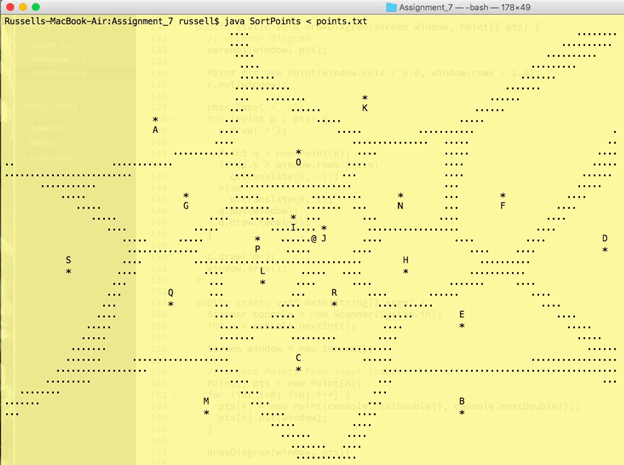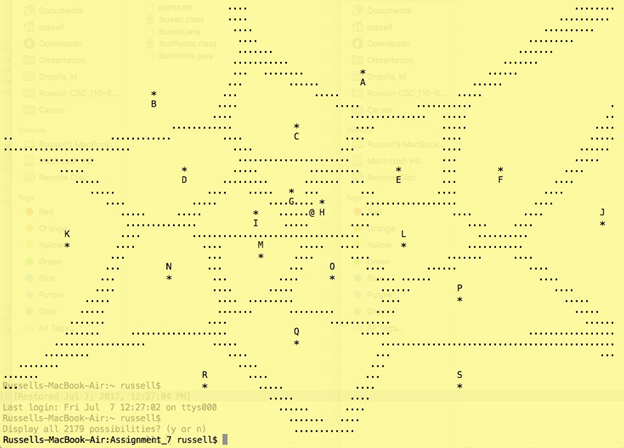# Assignment #7- SortPoints Solved

25.00 \$ 12.50 \$

Category:
Click Category Button to View Your Next Assignment | Homework

You'll get a download link with a: . ` zip` solution files instantly, after Payment

# Learning Outcomes

When you have completed this assignment, you should understand:

• How to use custom Classes in a client program.
• How to read a file in to a program from the command line.
• How to write Selection Sort code.
• How to write Bubble Sort code.
• How to change a sorting of elements based on your own comparisons.
• How to create a voronoi diagram.
• (That you can use different measures of distance from Euclidean distance.)

Download the template program file SortPoints.java, along with the Screen.java and Point.java classes (they must be the ones from the Assignment 7 section on conneX). SortPoints.java has comments throughout that will help you complete your work. When finished the program SortPoints.java should read in a set of points from a file called points.txt (available for download on conneX) using the command line in your console. You can do this with the following command:

java SortPoints < points.txt

and you can read the values in the file using a Scanner connected to System.in. DO NOT use a File object. The marker will not run your program correctly if you do, and you will not receive full marks. The first value in points.txt is the number of (x,y) coordinates in the file, and you should save this value as an int in your program. Use it to create an array of Point objects with the appropriate number of elements, and then scan in the remaining values to create each Point in the array.

The (x,y) coordinates in points.txt are not listed in any particular order. The first code you should write will be part of the drawDiagram method. Make it draw the points on a Screen and label each Point in the order they are listed in your array from A to S (there are 19 of them). You can use a char variable and increment it as you label each Point. Use a copy of each Point to draw the label and change its (x,y) coordinates so that if the Point is above the centre of the Screen then the label draws directly below the Point, and otherwise the label should draw directly above the Point. Also, draw an @ character at the centre of the Screen object. Your output should look exactly like the output given below:You have been given the code for the dist method that calculates the distance in a slightly different way than the usual Euclidean distance formula you are used to. The only difference here is that the vertical distance has been scaled to contribute 3 times as much as normal. I’ve done this because the Screen object is defined to have roughly three times as much width as there is height and the Point objects are arranged in an elliptical spiral to match the aspect ratio of the Screen. There are actually many ways to consider calculating the distance between elements in a set such as points in the 2D plane, and Metric Spaces is a topic in mathematics that studies what happens when you define distances in different ways.

The next code you should write are two methods called voronoi and equidistant. The method equidistant has two parameters, an array pts of Point objects and another Point named p, and this method should check the distance of each Point in pts against p. Then if the two closest distances have an absolute value of their difference below 3.0 it should return true. Otherwise, it should return false. This code will be very much like finding the minimum, but you have to find the second to smallest value as well. (hint: look at the if/else code block inside the worley method of Animation.java in Lecture 15 code on conneX)

A voronoi diagram draws boundaries around a set of points, so that any position on a boundary is equidistant from its two closest points. These diagrams are useful for generating structures like cells, tiles, maps, etc.

The voronoi method should use nested for loops to iterate through every possible position in the Screen parameter and check whether each position is equidistant from its two closest Point objects in pts. If the position is equidistant, then it should set that position in the Screen to draw a period character. The drawDiagram method should call the voronoi method. Your output should look exactly like the output given below:Now you should write code for the distSort to sort the array pts so that they are labelled in the order of the closest to the centre of the Screen. You must implement the Bubble Sort algorithm to do this, and make the ordering use the distCompare method given. If two Point objects are out of order, then distCompare will return −1 and you then need to swap their locations in the array pts. Your Bubble Sort implementation must use the swap method, which you also need to write.

After you print the diagram in main, use your distSort method to sort the array pts and then print the diagram again. The additional output should look exactly like the output given below:You can see that the Point objects are labelled in the order of an elliptical spiral, increasing from the centre of the printed Screen.Make sure your program prints the above described three diagrams. Please submit your finished SortPoints.java file to conneX.